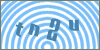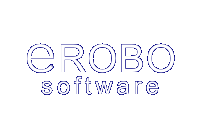Products
ServicesScripts: Cplus :: C++ string functions :: Library Article #3Developer's SectionConvert an Integer to a Binary Number in C++
By: Erobo Team Member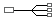Hire a Developer for Related Work / Installation | \$55 hr
 Rating: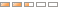| Rate It: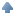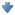Average Votes: (1259) Favorites:Code to convert an Integer to a String representing a Binary Numner.

This article shows how to convert any integer number to a string representing its corresponding binary value: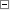Code Snippet 1//>>>>>>>>>>>>>>>>>>Converter<<<<<<<<<<<<<<<<<<<<<<
//method: convert to binary (unsigned)
string to_binary(int thisValue)
{

int comm = 0;

while( thisValue != 0 )
{
comm = (thisValue % 2);

if(comm == 0)

if(comm == 1)

thisValue = (thisValue / 2);
}See other Scripts in C++ string functionsSubmit Your Scripts:If you would like to have your C++ scripts published in this section please fill outthe form below:
 [ Refresh Image ]